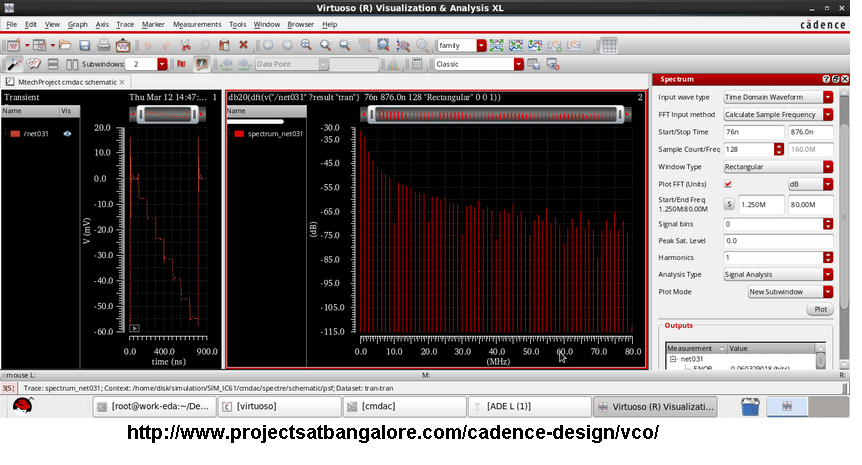Call:9591912372

VCO Design in CadenceCMOS VCO Design using Cadence## Voltage Controlled Oscillator | VCO

Voltage controlled oscillator (VCO), from the name itself it is clear that the output instantaneous frequency of the oscillator is controlled by the input voltage. It is a kind of oscillator which can produce output signal frequency over a large range (few Hertz-hundreds of Giga Hertz) depending on the input DC voltage given to it.

## Frequency Control in Voltage Controlled Oscillator

Many forms of VCOs are generally used. It can be of RC oscillator or multi vibrator type or LC or crystal oscillator type. However; if it is of RC oscillator type, the oscillation frequency of output signal will be in inversely proportional to capacitance.

## Types of Voltage Controlled Oscillator

The VCOs can be categorized based on the output waveform:

• Harmonic Oscillators

• Relaxation Oscillators

## Working Principle of Voltage Controlled Oscillator (VCO)

VCO circuits can be designed by means of many voltage control electronic components such as varactor diodes, transistors, Op-amps etc. Here, we are going to discuss about the working of a VCO using Op-amps. The output waveform of this VCO will be square wave. As we know the output frequency is related to the control voltage. In this circuit the first Op-amp will function as an integrator. The voltage divider arrangement is implemented here. Because of this, the half of the control voltage that is given as input is given to the positive terminal of the Op-amp 1. The same level of voltage is maintained at the negative terminal. This is to sustain the voltage drop across the resistor, R1 as half of the control voltage.
When the MOSFET is in on condition, the current flowing from the R1 resistor passes through the MOSFET. The R2 have half the resistance, same voltage drop and twice the current as that of R1. So, the extra current charges the connected capacitor. The Op-amp 1 should provide a gradually increasing output voltage to supply this current.

download cadence virtuoso for windows